### 世上没有如果，但有IF（狗头）——IF独立宣言。

1，认识IF函数

IF函数属于逻辑判断函数，它可以根据指定条件是否成立，返回对应的结果。官方简历如下：

IF(logical_test,value_if_true,value_if_false)

IF(喂~谈个条件吧，如果条件成立~怎么怎么着，如果条件不成立~又怎么怎么着)

=IF(B2>=60,"及格","不及格")

B2>=60是一个表达式，判断B2单元格的值是否大于等于60。

=IF(B2>=60,"不及格","及格")2，IF函数多层嵌套

=IF(B2<60,"不及格",IF(B2>90,"优秀","及格"))

……

=IF(B2>90,"优秀",IF(B2>60,IF(B2>80,"良好","及格"),"不及格"))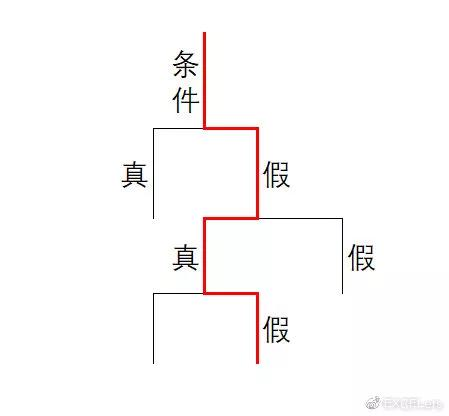=IF(B2>90,"优秀",IF(B2>80,"良好",IF(B2>60,"及格","不及格")))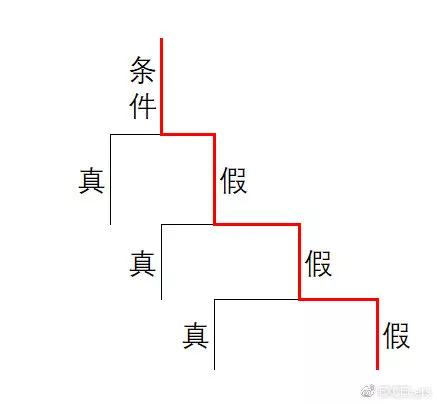……

IF函数简单又好用，但有句话不知当讲不当讲反正我要讲，当IF函数嵌套超过三层时，必然会有更高效更简洁的函数代替它工作。

### 3，AND和OR函数=AND(条件1，条件2，条件3，条件n……)

=AND(2>1,3>2,4>3)

AND函数包含了三个条件，每个条件计算结果都为TRUE，也就是说每个条件都成立，计算结果返回TRUE。

=AND(1>2,3>2,4>3)

……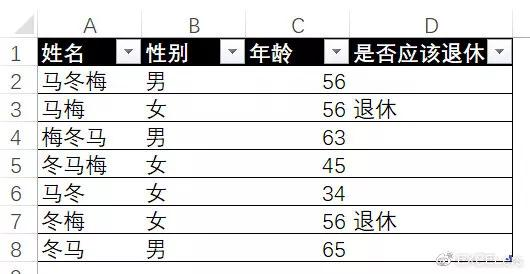D列公式如下：

=IF(AND(B2="女",C2>55),"退休","")

AND函数是一个表达式，作为IF函数的第一参数，有两个判断条件，一个是B2单元格性别是否为“女”，另一个是C2单元格的年龄是否大于55，只有两个条件都成立，AND结果才返回TRUE。

……

……

“或”关系刚好和“并且”关系唱反调，“并且”关系认为所有的条件都成立，才算数儿。“或”关系不这么认为，它觉得做人要大度，有一个条件成立就算OK了，只有所有条件都不成立时，才不算数。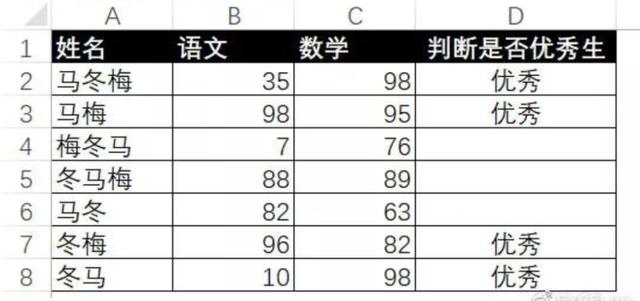D列公式如下：

=IF(OR(B2>90,C2>90),"优秀","")

OR函数是一个表达式，作为IF函数的第一参数，有两个判断条件，分别判断语文和数学的成绩是否大于90分，如果有一个条件成立，则OR函数返回TRUE。

……

……

=IF(60<B2<80,"良好","")

### 新人对于本节内容，可先了解，不用强求理解。……

……

= IF(1, TRUE, FALSE)，结果为TRUE，这意味着在IF函数眼中，1就等同于逻辑值TRUE。

= IF(0, TRUE, FALSE)，结果为FALSE，这意味着在IF函数眼中，0就等同于逻辑值FALSE。

= IF(-1, TRUE, FALSE)，结果为TRUE，这意味着在IF函数眼中，负数也等同于逻辑值TRUE。

……

AND函数是这样的：

=IF(AND(B2>90,C2>90),"优秀","")

=IF((B2>90)*(C2>90),"优秀","")

=(条件1)*(条件2)*(条件n)

……

OR函数是这样的：

=IF(OR(B2>90,C2>90),"优秀","")

=IF((B2>90) (C2>90),"优秀","")

=(条件1) (条件2) (条件n)

……

AND和OR能干的事，乘法和加法都能做。但乘法和加法能做的事，前者就不一定做的来了。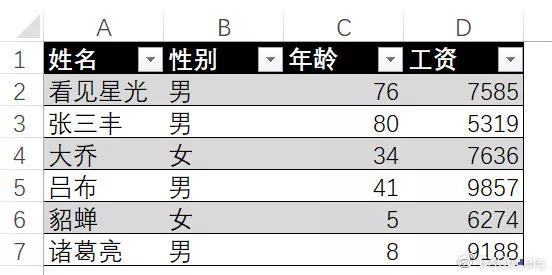=SUMPRODUCT((B2:B7="男")*(C2:C7>30)*D2:D7)

=SUMPRODUCT(AND(B2:B7="男",C2:C7>30)*D2:D7)

(B2:B7="男")*(C2:C7>30)返回的是一组值，比如此例中返回内存数组：{1;1;0;1;0;0}